# Compartment Models

12 November 2020 (Lecture 21)

# Previously

• Markov models: The future of the process is independent of its past, given its present state
• Everything boils down to the probabilities or rates of transitions between states
• Inference for Markov models: Based on the likelihood, or on other ways of assessing fit to the data
• Epidemic models: Every individual in the population is in one of a limited number of states of health; transitions between states happen at rates which depend on how many individuals are in each state

# Compartment Models

• Many sciences deal with populations, where each individual is in one of a limited number of states
• Epidemiology (as we saw)
• Ecology
• Sociology (think: occupations, classes, education, racial/ethnic groups, religion, …)
• Demography (age, sex, ethnicity, religion, …)
• Chemical kinetics (molecular “species”)
• All these use compartment models
• Compartments = status for individuals
• Each individual is in 1 and only 1 compartment at any time
• Compartments are often intersections, e.g., “Black males aged 61–65”
• Rates of transitions from one compartment to another, often depending on occupancy of compartments

# Basic Definitions and Assumptions

• Start with a “closed” population, constant size $$n$$
• Population is divided into $$r$$ compartments
• State of population at time $$t$$, $$X(t)$$, is a vector of counts, $\begin{equation} X(t) \equiv [X_1(t), X_2(t), \ldots X_r(t)] \end{equation}$
• $$X_i(t)=$$ how many individuals are in compartment $$i$$ at time $$t$$
• $$X(t)$$ changes because individuals move from compartment to compartment, randomly
• $$p_{ij}=$$ probability that individual in compartment $$i$$ will move to compartment $$j$$ at the next time step, the $$i\rightarrow j$$ transition rate
• If $$p_{ij} =0$$ the $$i\rightarrow j$$ transition is forbidden (or suppressed)
• $$p_{ii}=$$ probability of staying in compartment $$i$$
• Assumption: $$p_{ij}$$ is a function of the current state $$X(t)$$ and not the past or future states
• $$p_{ij}$$ might be constant for some $$i, j$$
• Assumption: All individuals in the population make independent transitions, given $$X(t)$$
• Transitions from compartment $$i$$ are independent and identically distributed, given $$X(t)$$
• These assumptions could be wrong!

# Consequences of the Assumptions

• $$Y_{ij}(t+1) =$$ number of individuals transitioning from compartment $$i$$ to compartment $$j$$ at time $$t+1$$, the flow or flux from $$i$$ to $$j$$
• By the assumptions, $\begin{eqnarray} Y_{ij}(t+1) & \sim & \mathrm{Binom}(X_i(t), p_{ij}(X(t)))\\ \mathbb{E}\left[ Y_{ij}(t+1)|X(t) \right] & = & X_i(t) p_{ij}(X(t))\\ \mathrm{Var}\left[ Y_{ij}(t+1)|X(t) \right] & = & X_i(t) p_{ij}(X(t))(1-p_{ij}(X(t))) \end{eqnarray}$
• Stock-flow consistency: $\begin{eqnarray} \label{eqn:stock-flow} X_i(t+1) & = & X_i(t) + \sum_{j\neq i}{Y_{ji}(t+1) - Y_{ij}(t+1)}\\ \text{(new level)} & = & \text{(old level)} + \sum{\text{(in flow)} - \text{(out flow)}} \end{eqnarray}$
• Implication: $$X(t)$$ is a Markov process
• Because $$Y_{ij}$$s only depend on current $$X(t)$$, not past
• The state space is finite, but very large

# Large-Population ($$n$$) Asymptotics

• We want to consider very large populations ($$n\rightarrow\infty$$) making lots of small steps over short time intervals $$h$$ ($$h\rightarrow 0$$) (so we go from time $$t$$ to $$t+h$$, not $$t$$ to $$t+1$$)
• Assume $$p_{ij}(X) = h \rho_{ij}(X/n)$$
• Meaning that the distribution over compartments matters, not the raw numbers in each compartment
• Define $$x(t) = X(t)/n$$
• As $$n\rightarrow\infty$$ and $$h\rightarrow 0$$, we go to deterministic differential equations, $\frac{dx_i}{dt} = \sum_{j \neq i}{\rho_{ji}(x(t)) - \rho_{ij}(x(t))}$
• There are some caveats — see the handout

# Epidemic Models

• SIR model from last time: 3 compartments, transition rates depend on counts in each compartment
• We’d now write $$X_S(t), X_I(t), X_R(t)$$ instead of $$S(t), I(t), R(t)$$
• $$Y_{SI}(t+1) = C(t+1)$$ from last time, $$Y_{IR}(t+1) = D(t+1)$$
• $$p_{SI} \propto X_I(t)$$, $$p_{IR} =$$ constant
• All other transitions are forbidden
• SIS model: 2 compartments, all transitions both ways
• SIRS model: 3 compartments, allow an $$R\rightarrow S$$ transition but no $$R\rightarrow I$$ or $$I \rightarrow S$$
• Often used for influenza

# Sociological Application: Modeling the Spread of Novelties

• Including: ideas, practices, technologies, use of products, beliefs, …
• Some practices spread by personal contact
• E.g., to learn how to make pots in a certain way, you (probably) need to learn from someone who already knows how to make that kind of pot
• Others can be spread by artifacts (you see a new kind of pot and can guess how to make it), or get re-invented
• Assume that everyone either has adopted the new practice (and can/will spread it), or that they haven’t
• $$\Rightarrow$$ 2 compartments, call them $$A$$ (adopters) and $$N$$ (non-adopters)
• Assume that the new practice spreads by personal contact
• So $$p_{NA}$$ depends on $$X_A$$, say $$\propto X_A$$
• Assume that no one gives up the practice, so $$p_{AN} = 0$$

• Assuming that the practice has to spread by personal contact:
• Some practices can be spread by artifacts
• Maybe you see a new-to-you type of pot and you can figure out how to make it
• Ideas can be spread by books, videos, etc., as well as by people
• Some practices get re-invented independently
• Not necessarily true by any means
• Maybe you just want to make pots and aren’t interested in teaching anyone
• Assuming the rate of adoption $$\propto X_A$$:
• Presumes some sort of random mixing within the population
• Presumes no independent adoptions (could add a constant term to the rate)
• Presumes no “diminishing returns” to encountering lots of adopters
• Presumes presumes there aren’t other influences on the decision to adopt or not
• Maybe un-common practices are always less attractive, because it helps to coordinate with others
• Assuming no one gives up the new practice, $$p_{AN} = 0$$:
• Plenty of people try new technologies, products, beliefs, etc., and then give them up!

$$\Rightarrow$$ Whether the assumptions are good matches to reality is something you need to investigate case by case, not presume!

# Comment: Analogy to Epidemic Models; What to Call This

• This is like an SIR model:
• Adopters $$A$$ $$\Leftrightarrow$$ Infectious $$I$$
• Non-adopters $$N$$ $$\Leftrightarrow$$ Susceptibles $$S$$
• But set $$p_{IR} = 0$$ (i.e., in terms of last time, $$\gamma=0$$)
• $$\therefore$$ The infectious stay infectious forever
• Some kind of analogy between the transmission of ideas/behavior and the transmission of disease is very old and very common
• E.g., Siegfried (n.d.) is a careful working-out of the analogy by a French geographer in 1960
• The mathematical models, and the realization that it matches epidemiological models, comes from the 1950s
• Talk about “diffusion of innovations” (Rogers 2003) and it sounds good
• Talk about “viruses of the mind” (Dawkins 1993) and it sounds bad
• It’s hard come up with a really neutral name
• The anthropologist Dan Sperber suggested “epidemiology of representations” (Sperber 1996), which I like but is a bit of a mouthful

# Consequences of the Model Assumptions

$\begin{eqnarray} Y_{NA}(t+1) & \sim & \mathrm{Binom}(X_N(t), \alpha X_A(t))\\ X_A(t+1) & = & X_A(t) + Y_{NA}(t+1)\\ X_N(t+1) & = & X_N(t) - Y_{NA}(t+1)\\ \mathbb{E}\left[ X_A(t+1)|X_A(t) \right] - X_A(t) & = & X_N(t)\alpha X_A(t)\\ & = & \alpha X_A(t)(n-X_A(t)) \end{eqnarray}$
• Notice: expected increment to $$X_A$$ is quadratic in $$X_A$$, small if $$X_A$$ is small, small if $$X_A$$ is big, maximized when $$X_A = n/2$$
• Notice: if $$X_A \ll n$$, then the increment is roughly $$\propto X_A$$, so initially there’s exponential growth
• “Slowly, and then all at once” but also “Nothing grows forever”

# Consequences of the assumptions

alpha <- 1e-6
n <- 1e5
xa <- vector(length=200)
xa <- 1
for (t in 2:length(xa)) {
xa[t] <- xa[t-1] + alpha*xa[t-1]*(n-xa[t-1])
}
plot(1:length(xa), xa, xlab="t", ylab=expression(X[A](t)), type="l")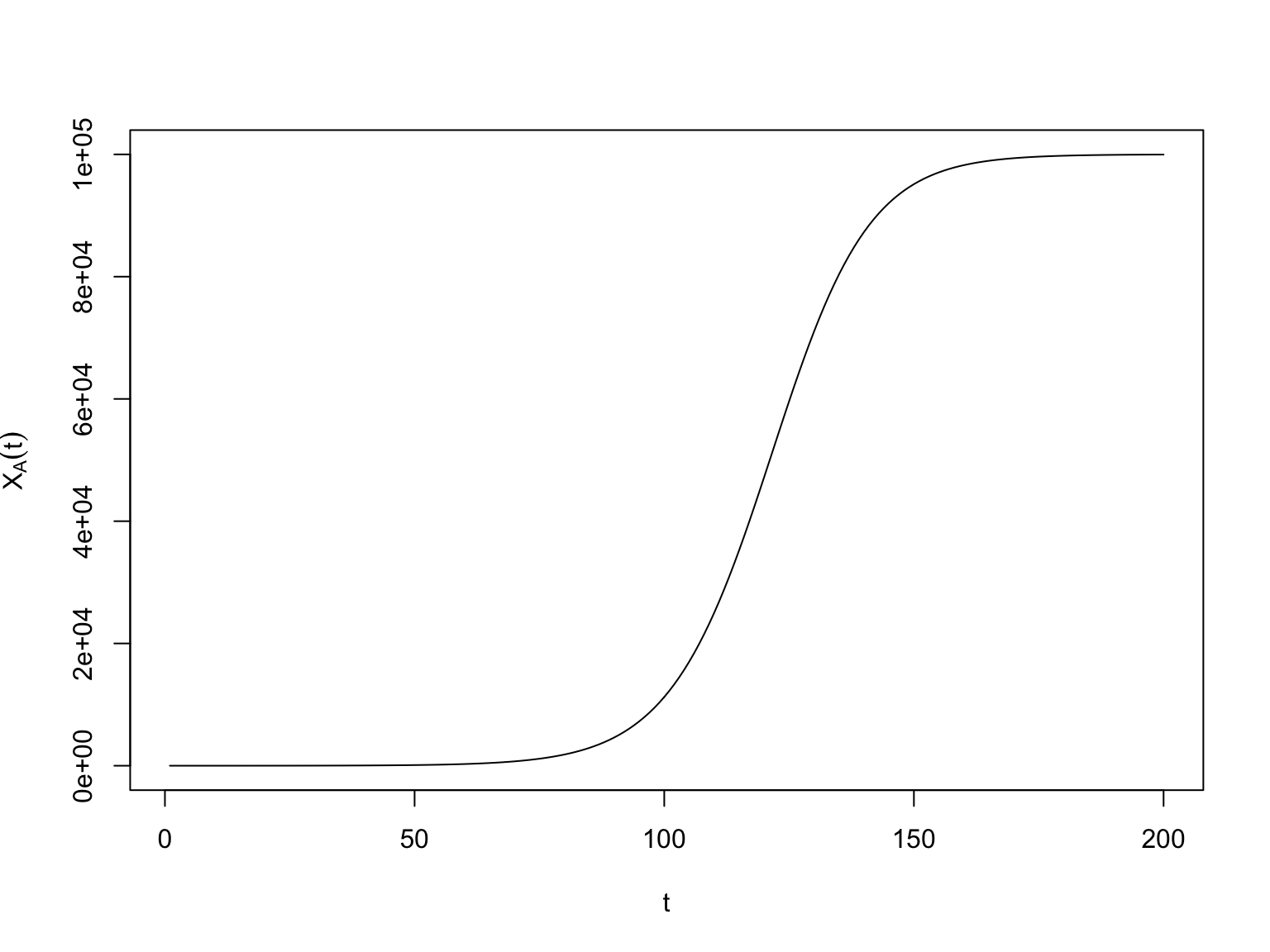• This looks like the logistic function we use in logistic regression, $$\frac{e^t}{1+e^t}$$
• This is, in fact, called a “logistic growth curve”
• Actually, the name “logistic regression” comes from this sort of logistic growth curve, not the other way around
• “Logistic” here from the sense of logistics, of supplying something (Bacaër 2011)

# Consequences of the assumptions

sim.diffusion <- function(n, alpha, xa.1=1, T) {
xa <- vector(length=T)
xa <- xa.1
for (t in 2:length(xa)) {
xa[t] <- xa[t-1] + rbinom(n=1, size=n-xa[t-1], prob=alpha*xa[t-1])
}
return(xa)
}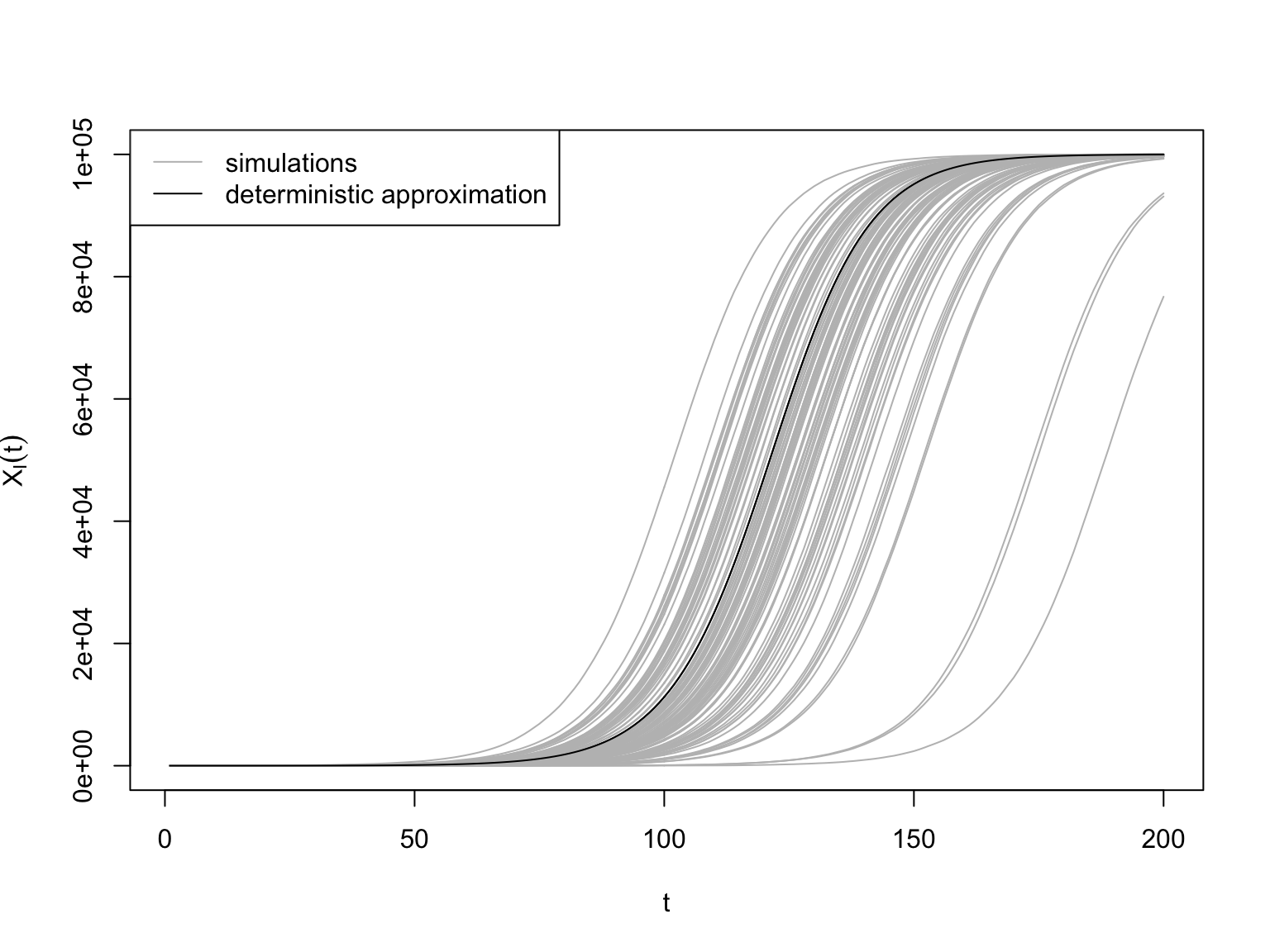# “Conversion to Islam in the Medieval Period”

• An interesting, important, and analytically-clean example from Bulliet (1979)
• Islam is a “missionary” religion; its adherents (often) go out to seek converts
• Some other religions aren’t missionary ones and conversion is rare or even impossible (Judaism, Shinto, etc.)
• Conversion requires personal interaction; you are converted by someone
• In some religions you might get converted just by reading (and believing) a book, but in all religions that’s rare
• During the initial expansion, say +630 to 900, lots of people converted to Islam
• Previously they were polytheists, Christians, Jews, Zoroastrians, Buddhists, Hindus, Manicheans, etc.
• There were many reasons for conversion — there were certainly practical advantages if living under Islamic rule — but part of it was definitely sincere religious conversions
• Hodgson (1974), Bulliet (1994), Ansary (2009) all try to present why people might’ve wanted to convert to Islam
• Bulliet (1979) used medieval biographical dictionaries to determine when many different conversions happened, in different countries
• The biographies give the subject’s dates, and their formal name, in the style “A, son of B, son of C, son of D, … son of Z”
• Converts typically assumed a Muslim name
• Tracking back to the latest non-Muslim name in a genealogy gives the number of generations to conversion
• Especially clear for Persian-speaking countries (modern Iran, Afghanistan, Uzbekistan, etc.) where the pre-Islamic names were linguistically very different from Arabic names, and also there weren’t many Jews and Christians with Biblical names (which are also common in Islam)
• Assuming so many years per generation says (roughly) when the conversion happened
• Bulliet grouped by 25-year intervals

# “Conversion to Islam in the Medieval Period”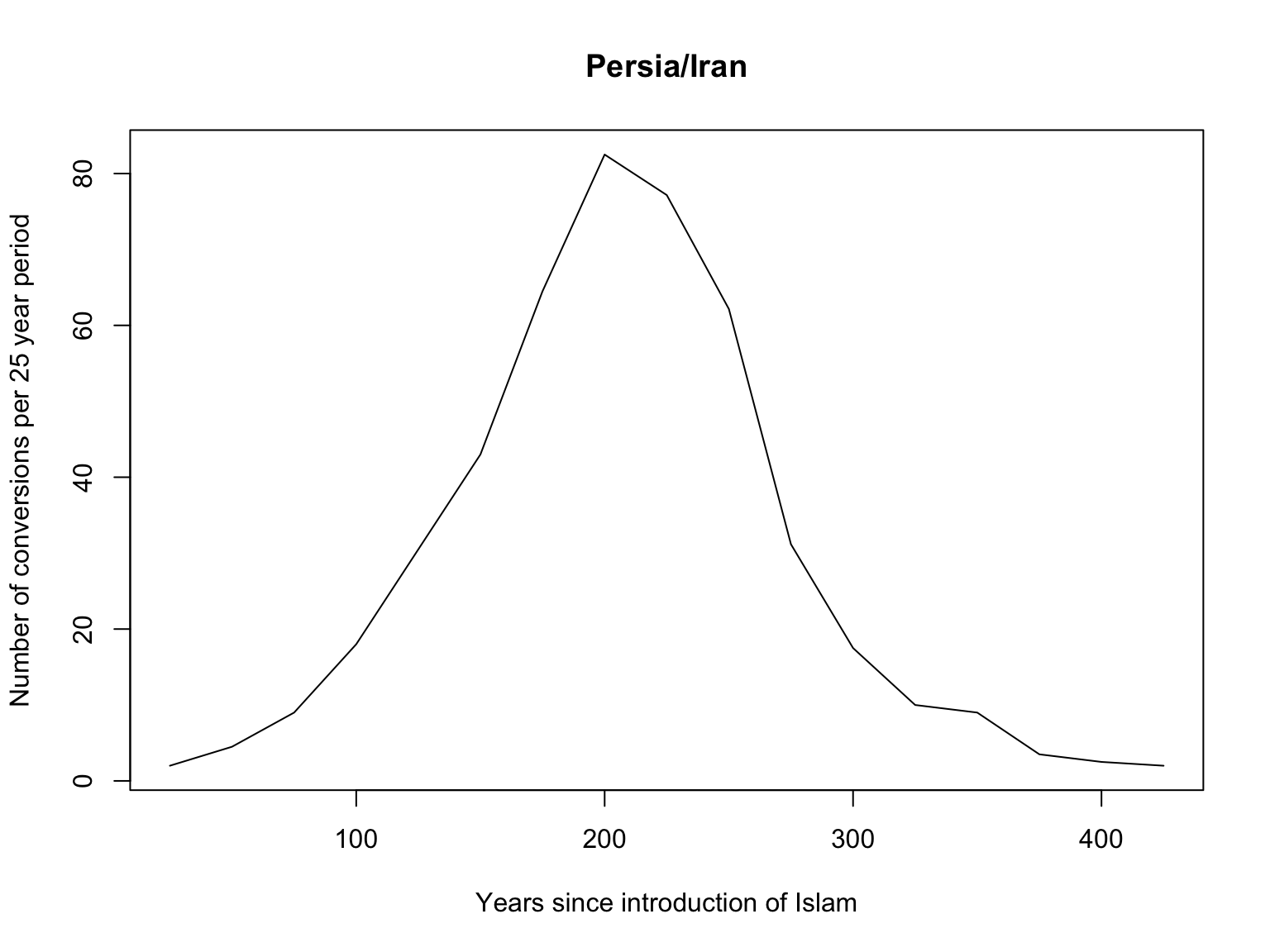# “Conversion to Islam in the Medieval Period”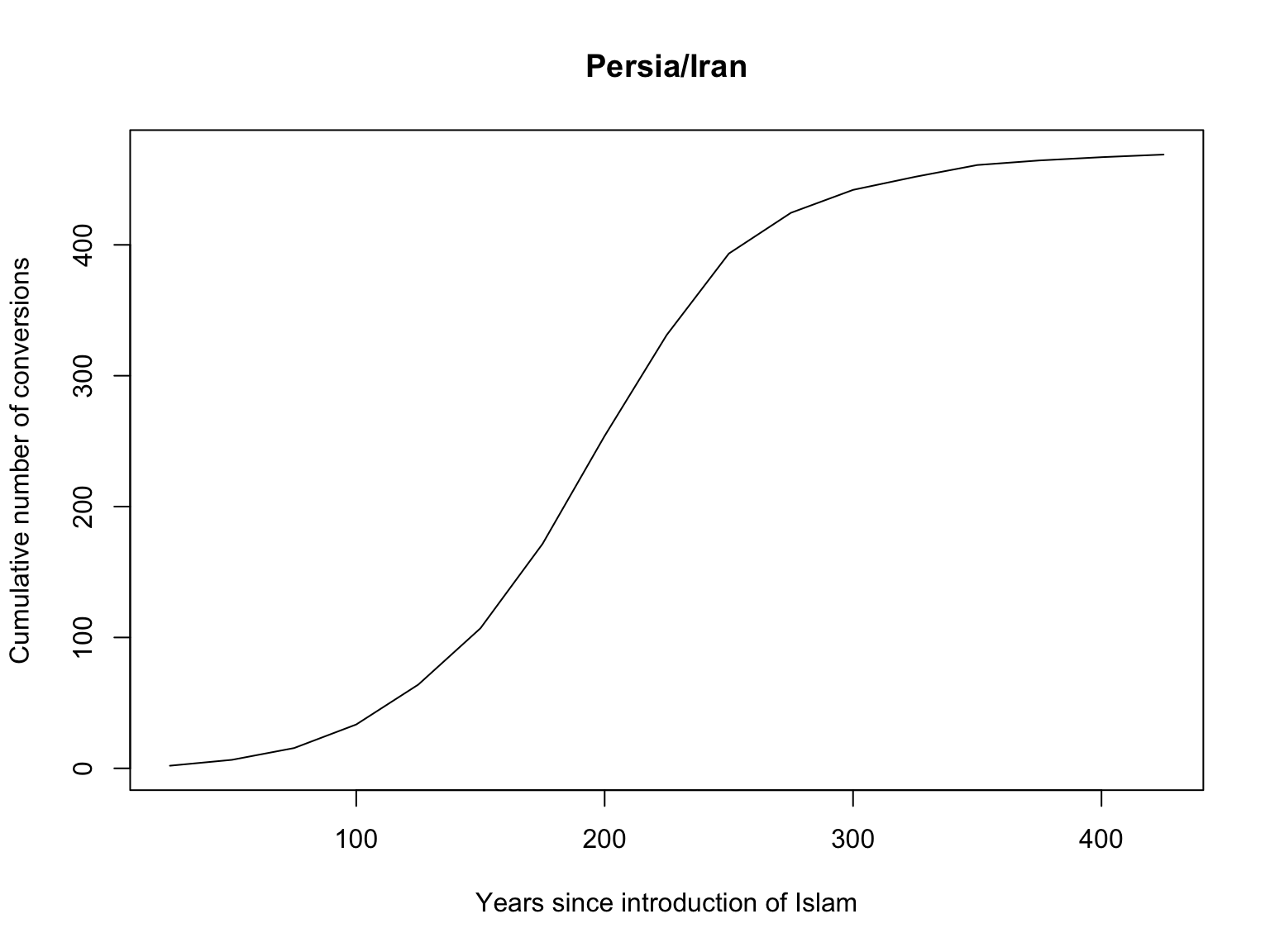• How could we fit the model to this data?
1. Regress the increments $$X_A(t+1) - X_A(t)$$ on $$X_A(t)(n-X_A(t))$$
2. Fit the curve $$X_A(t)$$ to the deterministic curve implied by the model
3. Use maximum likelihood

# “Conversion to Islam in the Medieval Period”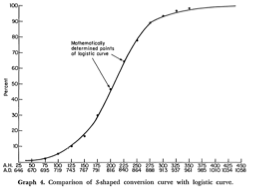# Summary

• Divide the population into mutually exclusive and jointly exhaustive compartments
• Assume both that transition rates between compartments only depend on the present state of the population, and that individuals transition (conditionally) independently
• Result: Markov model for the state of the population, with stable large-$$n$$ behavior
• Applications: epidemiology, spread of new ideas/practices, lots of other social processes, demography, …